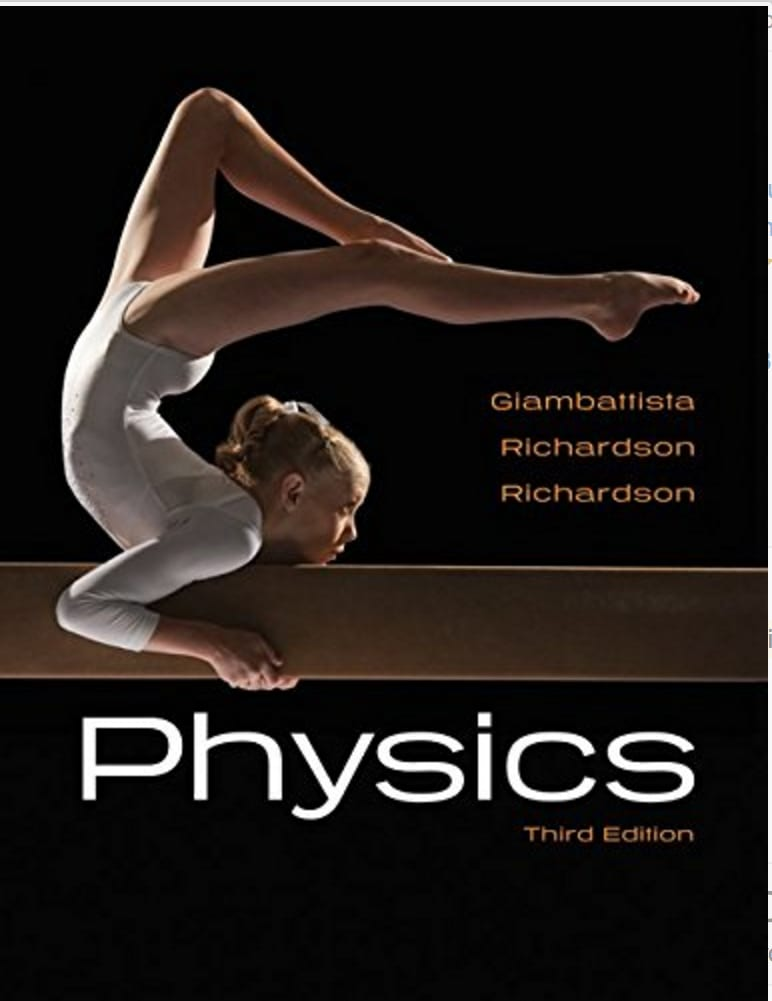Physics Physics# Physics Giambattista • 3rd Edition • 978-0073512150

Not the textbook you were looking for? Pick another one here.

## Ch 01: Introduction

Introduction to Units Unit Conversions Dimensional Analysis Counting Significant Figures Operations with Significant Figures

## Ch 03: Motion in a Plane

Intro to Vectors (Basic Trigonometry) Vectors with More Trigonometry Intro to 2D Motion Projectile Motion More Projectile Motion Initial Velocity in Projectile Motion

## Ch 10: Elasticity and Oscillations (NEW)

Spring Force (Hooke's Law) Intro to Simple Harmonic Motion (Horizontal Springs) Energy in Simple Harmonic Motion Simple Harmonic Motion of Vertical Springs Simple Harmonic Motion of Pendulums Energy in Pendulums

## Ch 10: Elasticity and Oscillations

Intro to Springs (Hooke's Law)

## Ch 11: Waves

What is a Wave? The Mathematical Description of a Wave Waves on a String Wave Interference Standing Waves

## Ch 12: Sound

Sound Waves Standing Sound Waves Sound Intensity Beats The Doppler Effect

## Ch 13: Temperature and the Ideal Gas

Temperature Zeroth Law of Thermodynamics Thermal Expansion Introduction to Ideal Gasses Kinetic Theory of Gasses

## Ch 15: Thermodynamics

First Law of Thermodynamics Thermal Processes Refrigerators Entropy and the Second Law Statistical Interpretation of Entropy

## Ch 20: Electromagnetic Induction

Intro to Induction Magnetic Flux Faraday's Law Lenz's Law Motional EMF Transformers Mutual Inductance Self Inductance Inductors LR Circuits

## Ch 22: Electromagnetic Waves

What is an Electromagnetic Wave? The Electromagnetic Spectrum Energy Carried by Electromagnetic Waves Electromagnetic Waves as Sinusoidal Waves Polarization Filters

## Ch 23: Reflection and Refraction of Light

Ray Nature Of Light Reflection Of Light Refraction Of Light Total Internal Reflection Refraction At Spherical Surfaces Ray Diagrams For Mirrors Mirror Equation Ray Diagrams For Lenses Thin Lens And Lens Maker Equations

## Ch 25: Interference and Diffraction

Diffraction 36.02 (A, n/a): Diffraction with Huygen's Principle Young's Double Slit Experiment Single Slit Diffraction

## Ch 26: Special Relativity

Inertial Reference Frames Special Vs. Galilean Relativity Consequences of Relativity Lorentz Transformations